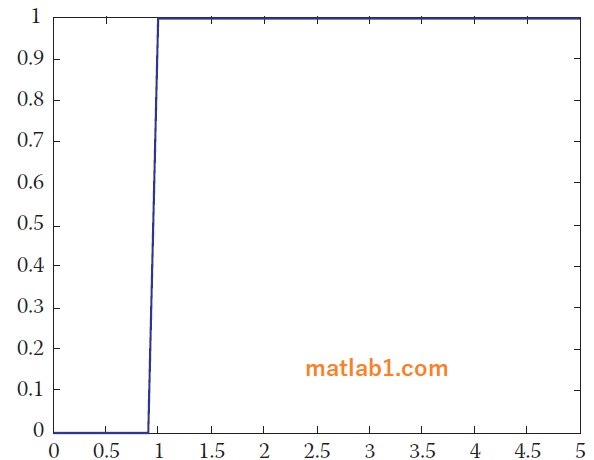MATLAB provides built-in functions for unit step function u(t) and unit impulse function δ(t). The unit step function is called Heaviside or stepfun, while the impulse function is Dirac. Heaviside(t) is zero when t < 0, 1 for t > 0 and 0.5 for t = 0. stepfun(t,t0) returns a vector of the same length at t with zeros for t < t0 and ones for t > t0. stepfun(n,n0) works the same way for discrete signals. Dirac(t) is zero for all t, except t = 0, where it is infinite.

MATLAB treats continuous-time signals differently from discrete-time signals. We will treat them separately in the following example.

The step function starting at t = 1 and going right :

The MATLAB script for this case is presented as follows:

```t = 0:0.1:5
t0 = 1;
u = stepfun(t,t0);```